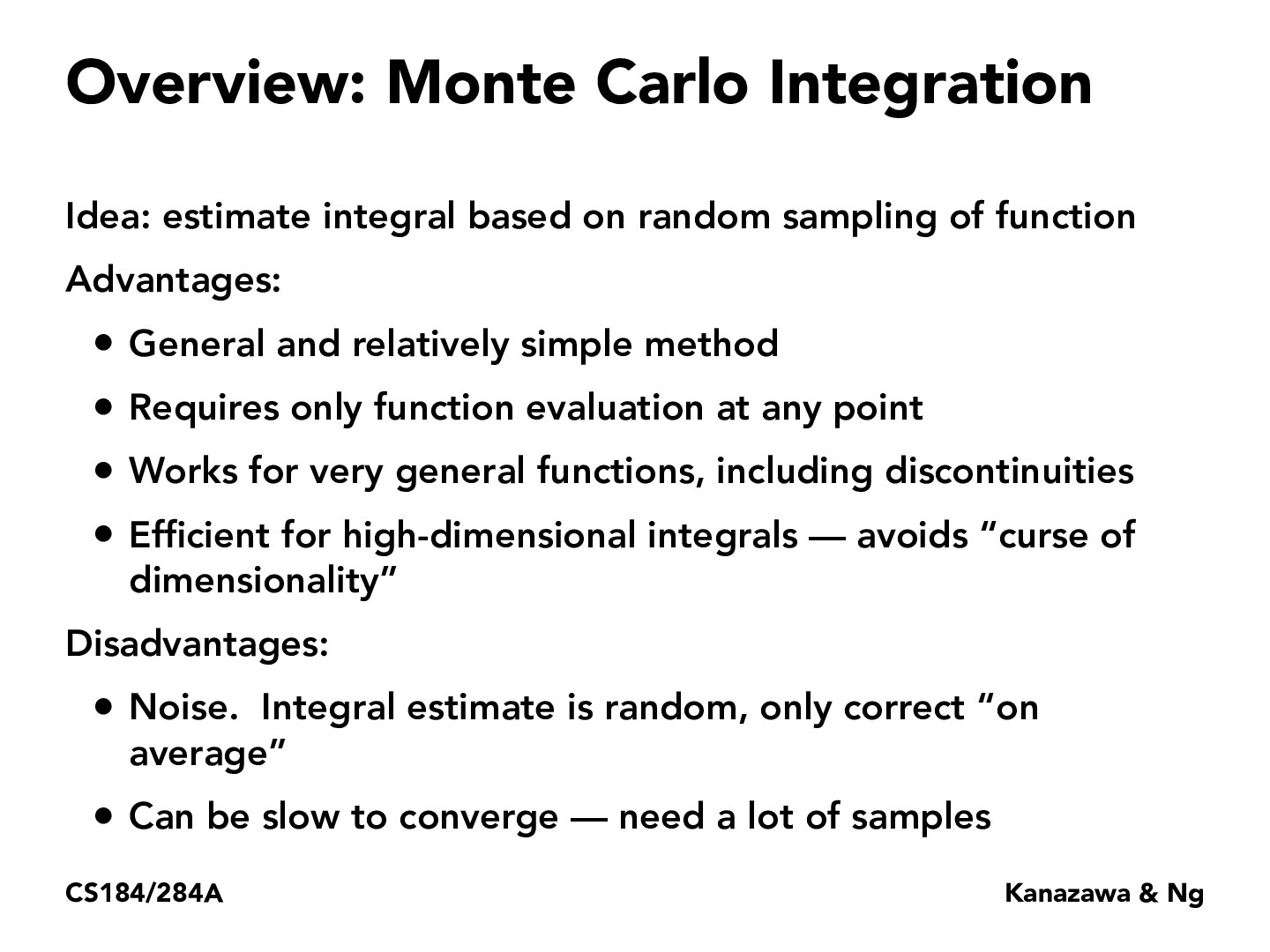Lecture 12: Monte Carlo Integration (12)bveeramani

I'm confused as to why "slow to converge" is listed as a disadvantage for Monte-Carlo integration. Monte-Carlo integration avoids the curse of dimensionality, so I assume that Monte-Carlo integration requires fewer samples than quadrature-based integration. If Monte-Carlo integration requires fewer samples than quadrature-based integration, wouldn't it make more sense to list the convergence rate as an advantage?philippe-eecs

I think it is mainly much much faster than quadrature-based integration, but since we integrate over a region we'd need a ton of samples to get a full picture. Since calculus is a continuous mathematic where small changes make a big difference, you'd lots of samples to fully define your problem. So each sample we take must represent an important portion of our region of integration.pixelled

I think "Can be slow to converge" here refers to the convergence of error in Monte Carlo integration. Even though its estimation should be unbiased, we still need enough number of random points to achieve as little error as possible. THerefore, the Monte Carlo integeration requires a lot of samples.ZizhengTai

I think there is similar usage of random sampling when we search for the optimal hyperparameters in deep learning. For complicated models, a random hyperparameter search is preferred over a grid search: https://stats.stackexchange.com/questions/160479/practical-hyperparameter-optimization-random-vs-grid-searchdidvi

This is an interesting comparison @ZizhengTai, do they use monte carlo integration as a motivation for using random sampling?

You must be enrolled in the course to comment